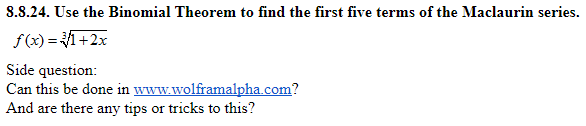8.8.24. Use the Binomial Theorem to find the first five terms of the Maclaurin seriesf(x):12.xSide question:Can this be done in www.wolframalpha com?And are there any tips or tricks to this?

Question

8.8.24. Use the Binomial Theorem to find the first five terms of the Maclaurin series.help_outlineImage Transcriptionclose8.8.24. Use the Binomial Theorem to find the first five terms of the Maclaurin series f(x):12.x Side question: Can this be done in www.wolframalpha com? And are there any tips or tricks to this? fullscreen
Step 1

The bonomial expansion of (1+y)^n , where n is any index is shown on board.

Step 2

Now, we substitute y=2x and n=1/3 to...

Want to see the full answer?

See Solution

Want to see this answer and more?

Our solutions are written by experts, many with advanced degrees, and available 24/7

See Solution
Tagged in

Other